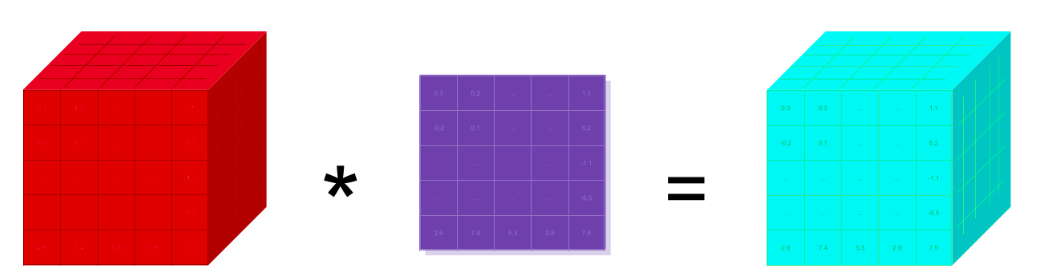zero

• 5

获得赞
• 2

发布的文章
• 0

答辩的项目

## PyTorch学习笔记（二）：关于张量的阶、轴和形状的解释

PyTorch

PyTorch

• 形状

• 我们有一个矩阵
• 我们有一个二维数组
• 我们有一个二维张量

A tensor's rank tells us how many indexes are needed to refer to a specific element within the tensor.

An axis of a tensor is a specific dimension of a tensor.

```t
t
t```

```t
t
t

t
t
t

t
t
t

t
t
t```

`dd = [[1,2,3],[4,5,6],[7,8,9]]`

```dd
[1, 2, 3]

dd
[4, 5, 6]

dd
[7, 8, 9]```

```> dd
1

> dd
4

> dd
7

> dd
2

> dd
5

> dd
8

> dd
3

> dd
6

> dd
9```

The shape of a tensor gives us the length of each axis of the tensor.

`> dd = [[1,2,3],[4,5,6],[7,8,9]]`

```> t = torch.tensor(dd)
> t
tensor([[1, 2, 3],[4, 5, 6],[7, 8, 9]])

> type(t)
torch.Tensor```

```> t.shape
torch.Size([3,3])```另外，当我们对神经网络进行编程时，我们必须经常执行的一种操作叫做reshape。 当我们的张量在网络中流动时，在网络内部的不同点上会出现特定的形状，作为神经网络程序员，我们的工作就是理解传入的形状，并有能力根据需要重构形状。

Shape 6 x 1

• number
• scalar
• array
• vector
• 2d-array
• matrix

Shape 2 x 3

• number, array, 2d-array
• scalar, vector, matrix

Shape 3 x 2

• number, scalar
• array, vector
• 2d-array, matrix

```> t = torch.tensor(dd)
> t
tensor([[1, 2, 3],[4, 5, 6],[7, 8, 9]])```

```> t.reshape(1,9)
tensor([[1, 2, 3, 4, 5, 6, 7, 8, 9]])

> t.reshape(1,9).shape
torch.Size([1, 9])```

```3 * 3 = 9
1 * 9 = 9```

Reshaping changes the shape but not the underlying data elements.500字zero

• 5

获得赞
• 2

发布的文章
• 0

答辩的项目